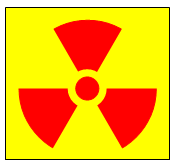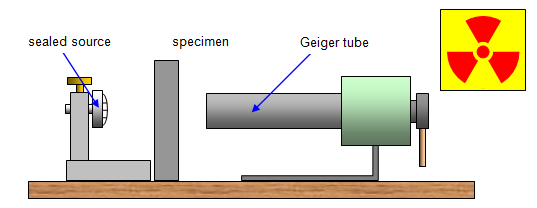In 1896 Henri Becquerel, who was Professor of Physics at the University of Paris, discovered that uranium salts could fog a photographic plate even if the plate was covered. He deduced that the salts were emitting some form of radiation that could pass through the wrapping. He also found that this radiation could ionise a gas, causing a charged electroscope to discharge.

In the same year Marie Curie and Schmidt discovered that thorium also produced this radiation.In 1898 Marie Curie extracted a much more active material from the ore pitchblende, which is 80 per cent uranium oxide. She called the radiation radioactivity and the active material radium. Radium is so active that its compounds stay a few degrees above the temperature of their surroundings.

In 1900 the radioactive gases thoron and radon were discovered by Rutherford and Dorn.

## Properties of the radiation

It was later realised that this radiation:
(a) came from the nucleus of unstable atoms
(b) was spontaneous – once material containing these unstable nuclei was formed emission could take place immediately and without the addition of other types of energy
(c) was random – it was impossible to predict which nucleus would emit radiation

Research showed that there were three different types of radioactivity and these were denoted by the Greek letters alpha(α), beta(β) and gamma (γ). The table below sets out the main properties of these radiations.

 Alpha radiation Beta radiation (MeV) Gamma radiation Penetration small large very large Range in air few cm tens of cm many metres Charge +2 +1 or -1 0 Deflection by electric or magnetic fields some large none Ionisation 10000 1000 1 Velocity 0.05c 0.9c c Mass 8000 1 '0' Nature helium nuclei electrons e.m radiation

The energy of a radioactive particle is usually expressed in million electron volts (MeV);

Since electrical energy = charge x voltage an electron volt is defined as the energy gained by an electron when accelerated through a potential difference of 1 volt and therefore:

1MeV is the energy acquired by an electron when accelerated through a potential difference of one million volts.
1 MeV = 1.6x10-13 J

## The absorption of radiation

In a vacuum the range of either alpha, beta or gamma radiation is infinite, since no ions are produced. The intensity of gamma radiation decreases with distance, following the inverse square law since it is electromagnetic radiation.

Alpha particles have a very short range in materials and their range is difficult to measure. The absorption of gamma radiation can be studied quite easily, however, and it is found that the intensity of the gamma radiation (I) varies with distance (t) through the material, according to the equation:

Gamma ray absorption:     log I = a - μt

where a and μ are constants for the material.

Student investigation
The absorption of gamma-radiation is of crucial importance in the nuclear power industry and this investigation is designed to measure this.
(a) Absorption in air
Set up the Geiger tube as shown in the diagram, and compare the count rates at different distances from the source.
Plot a linear graph of the results.(b) Absorption in building materials and soil
Prepare a suitable series of absorbers of concrete, brick and soil. Using the Geiger tube and scaler as before, measure the intensity of the gamma-beam with different thicknesses in place. Plot linear graphs relating count rate to absorber thickness, and deduce the thickness of each absorber required to reduce the gamma-ray flux to one-tenth of the original value.

Observe the normal radioactivity safety procedures when carrying out these investigations.

© Keith Gibbs 2013